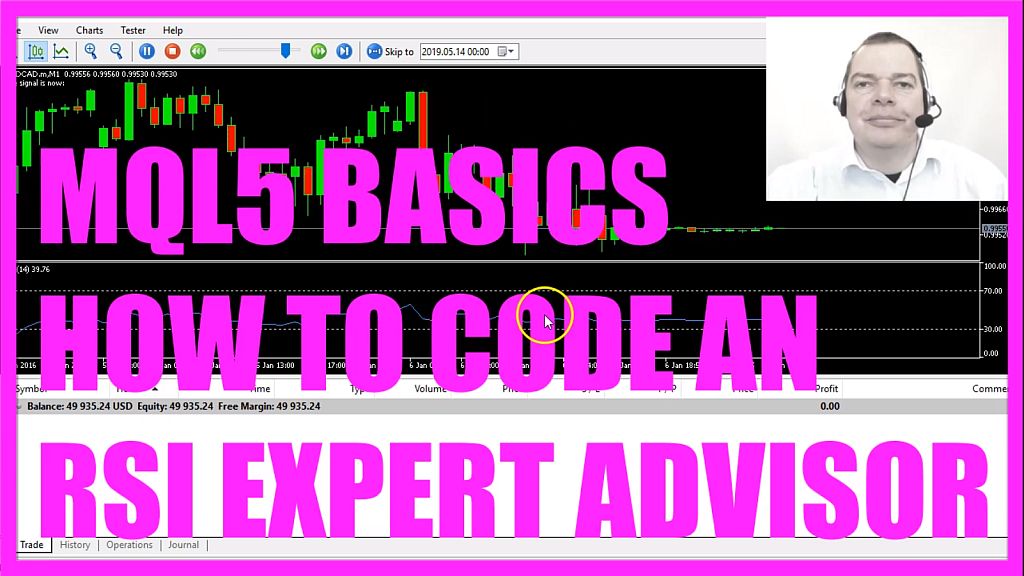In this video, we are going to create an Expert Advisor to automatically calculate and trade the Relative Strength Index also called RSI, so let’s find out how to do that with MQL5.
First you need to click on the little button here or press F4 on your keyboard, now you should see the Metaeditor window and here you want to click on: “File/ New File/ Expert Advisor (template)” from template, “Continue”, I will call this file: “SimpleRSIEA”, click on “Continue”, “Continue” and “Finish”.
Now you can delete everything above the “OnTick” function and the two comment lines here.
We start with an include statement, we want to include the file: “Trade.mqh”, it comes with trading functions and it is included in MQL5, so now we can create an instance called: “trade”.
Inside of the “OnTick” function we want to calculate the Ask price and the Bid price because we are going to buy and sell, so we use “SymbolInfoDouble”, “_Symbol“, “SYMBOL_ASK” to calculate the Ask price and with “SymbolInfoDouble”, “_Symbol“, “SYMBOL_BID” – all in capital letters – we will get the Bid price.
“NormalizeDouble” and “_Digits” is a way to automatically calculate the number of digits behind the dot because the “_Digits” variable stores the number of digits after the decimal point that might be 3 digits – like in this case – or 5 digits.
Let’s create a variable for the signal, the name is also “signal”, please don’t assign a value right now because we need to calculate that.
We need to create an array for the price data that will be called: “myRSIArray”, it’s a double array, so let’s define the properties of the RSI (myRSIDefinition), we have an included function in MQL5 that is called: “iRSI”, it takes a few parameters;
The first parameter is for the current symbol on the chart,
The second one for the period,
This value; 14, is also what you see if you click on: “Insert/ Indicators/ Oscillators/ Relative Strength Index”, here you have a period value for 14 candles and it is applied to the close prices.
This is how the Indicator looks like.
Until the last major update, it was automatically shown on the chart whenever I calculated it, but now I need to right-click into the chart, select “Templates/ Save Template” and save it as: “tester.tpl” because this is the template that is going to be used in the backtest and the default template is the one that you will see when you drag any Expert Advisor on the chart, so let’s choose “tester.tpl” and save it.
Yes, I want to override it, so this parameter is for the period,
This one is to calculate values based on the close price.
Now I use “CopyBuffer” to fill the price array (myRSIArray) that we have created here with data according to the definition (myRSIDefinition) that we made here.
This parameter here stands for the buffer. The buffer is in our case the blue line, this parameter here stands for the current candle 0 (zero), we want to calculate the values for 3 candles and store them in the RSI array (myRSIArray) and that will make it possible to calculate an RSI value (myRSIValue) for the current candle by simply looking into candle 0 (zero) of our RSI array (myRSIArray), “NormalizeDouble” and comma 2 is used to cut the output to two digits like in the Indicator and if the RSI value(myRSIValue) is bigger than 70 – that’s the case when it crosses this line – and is above we consider the value to be overbought, so we want to assign “sell” to our signal.
In the other case if the RSI value(myRSIValue) is below 30 we want to buy because when it’s below this line we think that it is oversold.
So if the signal equals “sell” and if “PositionsTotal” is below 1 – so we have no open positions – we want to use “trade.Sell” to sell 10 micro lot.